### Home > CC1 > Chapter 8 > Lesson 8.1.2 > Problem8-28

8-28.
1. Rewrite each expression below using the Distributive Property. Then evaluate each expression for x = 2 and y = 5. Homework Help ✎

1. 8x − 4

2. 6y2 + 24y

3. 7x(y − 2)

4. 3(9 + y)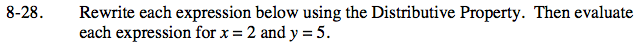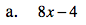What is a common factor of both terms?

Factor out a 4 from both terms.

4(2x − 1)

Substitute in the known values.

4(2(2) − 1)

Simplify.

12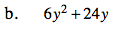Follow the steps in part (a).

270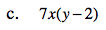Multiply what is in the parentheses by what lies on the outside.

7xy − 14x

Substitute in the known values.

7(2)(5) − 14(2)

42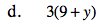Follow the steps in part (c).